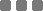## Wednesday, August 23, 2017

### JavaScript: How to Convert One Number Base (Radix) to Another

Basic: Decimal to Binary

For instance, we have number `7`, which is base-10 number. Then, we need to convert to base-2 (binary) system.

The base-2 system uses the addition of power of 2's to get the number we have in another radix system. So, to get the value from the other base system (base-10 for example), we continuously divide that number with 2, and take the remainder of each division.

In paper and pen scribbling math, we do this:

7 : 2 -> closest multiplier is 3, remainder 1

3 : 2 -> closest multiplier is 1, remainder 1

1 : 2 -> the only multiplier is 0, remainder 1

Put all remainders as a number, take each from the BOTTOM: 1 ► 1 ► 1

So, 710 (decimal) = `1112` (base-2 or binary system)

Or, we could use the addition method of power of 2:

\$\$ 7 = (\bo1 × 2^2) + (\bo1 × 2^1) + (\bo1 × 2^0)\$\$

Look at the multiplier (1), read it from the LEFT: 1 ► 1 ► 1

Meaning, again, 710 (decimal) = `1112` (base-2 or binary system)

JavaScript: Decimal to Binary

Use `toString(2)` to convert the base-10 (decimal) input to base-2 (binary) system. Hence, the 2 as argument's value in that `toString()` function. The output is `String` type.

Example:

To convert the output to number, use `Number()`. Like so:

This method also works for negative number.

For floating number (number with decimal point), this method will also work (but with some limitation for the radix point, the approximation).

Example:

JavaScript: One Base to Another Base

Basically, we implement `toString()` to convert one base to another:

BUT, that only works for decimal input. For other base input, we need one preliminary step to convert it to its actual base, then we can convert it to another base.

It's using `parseInt(input, radix)` function.

The `radix` value ranges from 2 (base-2) to 36 (base-36).

For instance, converting base-16 (hexadecimal) 21 to decimal:

So then ► 2116 = 3310

Converting base-2 (binary) 11011 to base-8 (octal):

► 110112 = 338

Converting base-12 (dozenal) 322 to base-5 (pental):

► 32212 = 33135

JavaScript: Conversion Error

If the input cannot be converted, usually because of the input error, the output will produce `NaN`.

If the error because of the unsupported `radix` value in `toString`, the browser will throw `RangeError` exception.

JavaScript: Base Conversion Formula

You could also put preceding filter before `parseInt` to make sure the input is valid, or in between `parseInt` and `toString()` or something else you might like to put.

This should be wrapped in a function block, thus it will be easier to run over and over again.

For example, let's create a function named `radix_converter`. This function has 3 arguments: `input`, `from_base`, and `to_base`. It will return the value from that "base conversion formula" above:

That's All...

Oh wait, there's an application which can accommodate until base-62JavaScript: How to Convert One Number Base (Radix) to Another
https://monkeyraptor.johanpaul.net/2017/08/javascript-how-to-convert-one-number.html?m=0